## Introduction

The metrology of the resistance unit has been continuously progressing since the discovery that the transverse resistance of a two-dimensional electron gas (2DEG) in a perpendicular magnetic field is quantized at universal values RK/i, where RKh/e2 is the von Klitzing constant, h the Planck constant, e the electron charge and i an integer1. Using GaAs-based heterostructures to form the 2DEG, it has been possible to develop quantum Hall resistance standards (QHRS) reproducing RK/2 with a relative uncertainty down to 3 × 10−11 (ref. 2), as well as accurate low- and high-resistance QHRS based on arrays of Hall bars3 and QHRS adapted to the alternating current (a.c.) regime4. More recently, it has been considered by metrologists that an accurate QHRS could be developed in graphene5,6, possibly surpassing the usual GaAs-based ones.

The Dirac physics in monolayer graphene manifests itself by a quantum Hall effect (QHE)7,8 with Landau levels (LLs) at energies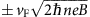with a 4eB/h degeneracy (valley and spin) and a sequence of Hall resistance plateaus at RHRK/(4(n+1/2)) (with n0)9. The energy spacing between the two first LLs,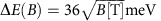, is much larger than in GaAs (1.7B[T] meV) for currently accessible magnetic fields. It results that the ν=2 Hall resistance plateau of value RK/2 (ν=hns/eB is the LL filling factor and ns the carrier density) can be observable even at room temperature10. This opens the way towards a more convenient QHRS in graphene5,11 operating at lower magnetic fields (B≤4 T), higher temperatures (T4 K) and higher measurement currents (I100 μA) compared with its GaAs counterpart. From previous measurements of the Hall resistance quantization in graphene produced by various methods (exfoliation of graphite, chemical vapour deposition (CVD) on metal and Si sublimation from SiC), it was concluded that the production of graphene-based QHRS (G-QHRS) requires large graphene monolayers (few 10,000 μm2) with homogeneous low carrier densities (<2 × 1011 cm−2) and high carrier mobilities (5,000 cm2 V−1 s−1). Thus, although the quantized Hall resistance was measured with a relative standard uncertainty of 6.3 × 10−9 (1 s.d.), on the ν=2 plateau at B=18 T and T=60 mK, in monolayer graphene obtained by mechanical exfoliation12, this technique was quickly discarded because it produces few monolayers of rather small size and it lacks reproducibility6,12,13,14. In the case of graphene grown by CVD on metal and transferred on a SiO2/Si substrate, the Hall resistance was measured far from being correctly quantized15,16. It was shown that the presence of grain boundaries and wrinkles jeopardized the quantization17,18. As far as we know, no accurate measurement of RH has been reported in graphene grown by CVD on metal. The quantized Hall resistance was measured by metrologists of the National Physical Laboratory in the United Kingdom (NPL), on the ν=2 plateau, in monolayer graphene grown by Si sublimation on the silicon face of SiC, produced by Linköping University. The agreement of the Hall resistance with RK/2 was demonstrated with a relative standard measurement uncertainty, down to 8.7 × 10−11 at B=14 T and T=0.3 K18,19, and slightly lower than 10−9 at B=11.5 T and T=1.5 K20. The NPL work also showed the flatness of the quantized Hall resistance plateau with a relative uncertainty of a few 10−9, over a 2.5 T range extending from 11.5 to 14 T20. In lower carrier- density samples produced by Graphensic AB, a spin-off from Linköping University research, the Hall resistance was measured on the ν=2 plateau at lower magnetic fields in the range from 2 to 8 T. However, the accuracy of the quantized Hall resistance was not demonstrated with a relative uncertainty better than a few 10−7 at 3 T and at 8 T21. Moreover, a large dispersion of the measurements (up to 0.5 × 10−6 in relative value) was observed by changing the Hall terminal pairs used, manifesting strong inhomogeneities in the samples. It turns out that no other 10−9-accurate QHRS (a QHRS accurate to within 10−9 in relative value) was achieved from any other graphene sources, although large-scale high-mobility graphene was produced22. Thus, only a few samples from a unique material supplier demonstrated the accuracy required in national metrology institutes, which compromises the sustainability of G-QHRS. Moreover, it was obtained under experimental conditions less convenient than those of currently used GaAs-based QHRS (GaAs-QHRS).

Here, we report on a G-QHRS made of monolayer graphene grown by propane/hydrogen CVD on SiC23, a hybrid technique that allows the tuning of the electronic transport properties24. The Hall resistance, measured on the ν=2 plateau with a 10−9 relative standard measurement uncertainty, is found in agreement with RK/2 over a 9 T-wide magnetic field range from B=10 T up to B=19 T at T=1.4 K. These cryomagnetic experimental conditions overlap those of the reference GaAs-QHRS used, making this device an operational QHRS substitute in current set-ups used in national metrology institutes. The relative discrepancy between the quantized Hall resistance of the G-QHRS and the GaAs-QHRS is found equal to (−2±4) × 10−10, which constitutes a new proof of the universality of the QHE. The QHE physics of the large ν=2 Hall resistance plateau is investigated using accurate measurement techniques based on specialized metrological instruments. It turns out that the dissipation is dominated by the variable range hopping (VRH) mechanism. The wide quantized Hall resistance plateau is characterized by a localization length of states at Fermi energy that remains very close to the magnetic length over a large magnetic field range of 9 T. This is likely caused by a pinning of the LL filling factor at ν=2 due to a charge transfer from the donor states in the interface layer between SiC and graphene. The measurement of a second 10−9-accurate G-QHRS fabricated from a different growth run, in similar cryomagnetic conditions as the GaAs-QHRS, establishes a worthy repeatability of the propane/hydrogen CVD on SiC growth method. Initiated in 2010 (ref. 23), this production technique is now mature and very promising to develop a challenging G-QHRS surpassing the GaAs-QHRS in the near future.

## Results

### Magnetoresistance characterizations

Figure 1b shows the Hall resistance RH and the longitudinal resistance per square Rxx measured as a function of the magnetic field B at a temperature T=1.4 K and a measurement current I of 100 nA in a large 100 × 420-μm Hall bar sample, inset Fig. 1b, made of graphene grown on the Si-face of SiC by propane/hydrogen CVD under a mixture of propane, hydrogen and argon23,25 (see Methods). At low magnetic fields, from the Hall slope and the Drude resistivity, a low electron density n0=3.2 × 1011 cm−2 and an electronic mobility μ=3,500 cm2 V−1 s−1 are calculated. At higher magnetic fields, a wide Hall resistance plateau RK/2 can be observed, from B=5 T up to B=19 T (maximum accessible magnetic field in our set-up) and coinciding with a dropping to zero of Rxx. It extends far beyond the magnetic field B=6.6 T at which ${v}_{{n}_{o}}\phantom{\rule{0.2em}{0ex}}\text{=}\phantom{\rule{0.2em}{0ex}}\text{2}$, where ${v}_{{n}_{o}}$ is the LL filling factor calculated from the carrier density determined at low magnetic fields. One can also notice the RK/6 Hall resistance plateau between 2 and 3 T. The comparison is striking when one compares the RK/2 Hall resistance plateau with the one of the most widespread in national metrology institutes GaAs-based QHR (LEP514 (ref. 26)), which only extends over 2 T starting from 10 T (green curve). Such robust QHE, characterized by a wide ν=2 Hall resistance plateau observable from low magnetic fields, were reproduced in other Hall bar samples fabricated from graphene grown by CVD on SiC, as discussed in Methods.Figure 1: Magnetic field dependence of the Hall quantization in graphene grown by CVD on SiC.

Figure 2 shows the colour rendering of the longitudinal resistance per square Rxx measured as a function of the measurement current I and the magnetic field B. It shows that the 2D electron gas is not significantly dissipative (Rxx<0.25 mΩ) for currents as high as 40 μA in the large range of magnetic fields between 10.5 and 19 T. We also carried out three-terminal measurements of contact resistances in the RK/2 Hall plateau. All contacts between metallic pads and graphene, except one (left terminal of the Hall bar, inset Fig. 1b), are ohmic with a resistance lower than 1 Ω. This is why in all the reported four-probes resistance measurements, the current circulates between I1 and I2 terminals. For a current of 20 μA, lower than the aforementioned limit of 40 μA, we performed accurate four-probe measurements of RH and Rxx (see Methods). Two Hall resistances are determined using Hall terminal-pairs (V1,V4) and (V2,V3). Two longitudinal resistances are determined using longitudinal terminal-pairs, located on both edges of the Hall bar, (V1,V2) and (V3,V5). Longitudinal resistances are normalized to a square.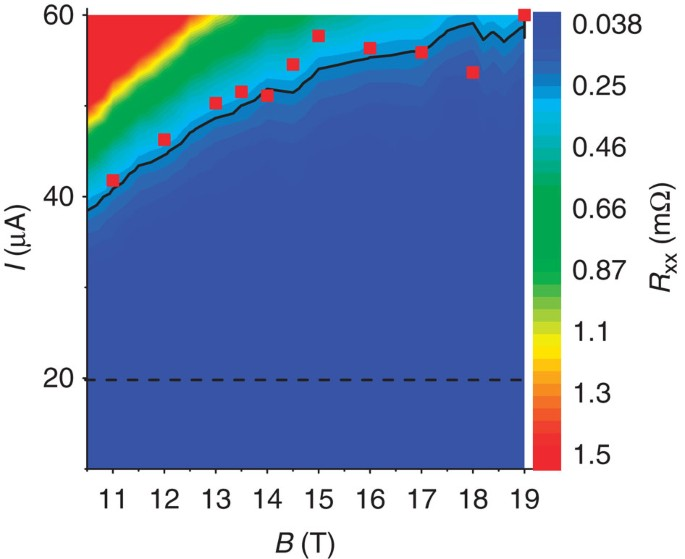Figure 2: Dissipation in (B, I) space in the ν=2 plateau.

### Resistance quantization

RH is indirectly compared with the RK/2 value given by a reference GaAs-QHRS (LEP514) using a 100 Ω transfer resistor. The comparison is performed using a resistance bridge equipped with a cryogenic current comparator (CCC). To minimize resistance comparison errors, the same measurement current and settings of the bridge are used to calibrate the 100 resistor either from the G-QHRS or the GaAs-QHRS. All uncertainties reported in the following are expressed as one 1 s.d. Let us note ΔRH/RH the relative deviation of the Hall resistance RH from RK/2 (ΔRH=RHRK/2).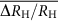and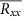are obtained from the mean value of the measurements performed using the two Hall terminal-pairs and the two longitudinal terminal-pairs previously mentioned. Figure 1a reportsvalues determined with a combined standard measurement uncertainty close to 1 × 10−9 (the main contribution comes from the instability of the 100-Ω transfer resistor) as a function of B for a measurement current of 20 μA and a temperature T=1.4 K. It shows a perfect quantization of the Hall resistance with no significant deviation over the whole magnetic field range of 9 T between B=10 T and B=19 T, which coincides with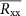values lower than (30±20) μΩ (see Fig. 1c):discrepancies are all within the expanded (k=2) standard measurement uncertainties (2 s.d.), where a coverage factor k=2 gives an expected confidence level of 95%. More sensitive measurements performed with the CCC (see Methods) show thatamounts to (10.5±2.4) and (1.2±1.7) μΩ at B=10 and 19 T, respectively, demonstrating a very low dissipation level in the graphene electron gas. Measurements show that the RH values determined from the two Hall terminal pairs are in agreement within a relative measurement uncertainty close to 1 × 10−9, demonstrating the homogeneity of the Hall quantization in the sample over a large surface. The mean value ofmeasurements carried out at magnetic fields between 10 and 19 T is −2 × 10−10 covered by an experimental s.d. of the mean of 4 × 10−10. Below B=10 T, the Hall resistance starts to deviate from the quantized value and the longitudinal resistance significantly increases. Figure 1a also reports thatis equal, at B=19 T, to (−1.5±0.4) × 10−9 and (−7±0.5) × 10−9 at T=2.2 and 4.2 K, respectively. This is due to an increase ofreaching 0.23 mΩ at T=4.2 K (see Fig. 1c). These Hall quantization measurements show first of all that the G-QHRS can operate accurately at magnetic fields as low as the ones of the reference GaAs-QHRS (10 T≤B≤11 T) with a similar temperature of 1.4 K. This demonstrates that this G-QHRS can directly replace a GaAs-QHRS in a conventional QHE set-up of a national metrology institute equipped with a 12-T magnet. The repeatability of the CVD on SiC growth method and of the technological process to obtain such competitive G-QHRS was tested by the measurement of another Hall bar device, having the same geometry, made of graphene produced in a different growth run (several months later). At T=1.3 K, the Hall resistance measured in this second G-QHRS device is also in agreement with RK/2 at B=10 T within a relative measurement uncertainty below 10−9 since ΔRH/RH=(4±8) × 10−10. As in the main G-QHRS, the flatness of the ν=2 Hall resistance plateau was demonstrated above 10 T over a magnetic field range larger than in GaAs-based devices: at B=10.8 T and at B=12 T, ΔRH/RH is found equal to (7±8) × 10−10 and (−2±8) × 10−10, respectively. This demonstrates a notable degree of reproducibility of the QHRS fabrication process, which is an asset for the resistance metrology application.

In the first presented G-QHRS, the relationship between RH(T) and Rxx(T) was investigated in the range from 4 to 40 K by performing measurements (using one Hall terminal-pair (V1, V4), and one longitudinal terminal-pair (V3, V5)) at magnetic fields between B=10 and 19 T using a low a.c. (2 Hz frequency) current of 1 μA. From measurements of both RH(T) and Rxx(T) (Fig. 3a), one can report ΔRH/RH(T) as a function of Rxx(T)/RH(T) for different B values in log-log scale (see Fig. 3b). The curves carried out at different B values are superimposed on a single straight line of unitary slope over four decades of Rxx. This corresponds to a relationship ΔRH=−0.67 × Rxx (see Fig. 3c). The same relationship is found by varying the magnetic field at a given temperature (not shown). We can therefore conclude that relative deviations of the Hall resistance from RK/2 are smaller than 10−9 for Rxx values lower than 15 μΩ. The longitudinal resistance values of (10.5±2.4) and (1.2±1.7) μΩ measured at B=10 and 19 T, respectively, should lead to small relative discrepancies to RK/2 of 6 × 10−10 and less than ≈1 × 10−10, respectively, thus experimentally not observable in Fig. 1. The linear relationship between RH and Rxx can be described by an effective geometric coupling. In GaAs-QHRS, this coupling is usually explained by the finite width of the voltage terminal arms with respect to the Hall bar channel5,27 or the inhomogeneous circulation of the current (for example, due to the residual inhomogeneity of the carrier density)28,29. The first mechanism would lead to a coupling factor of (−l/W)=−0.2, where l=20 μm is the width of the voltage arm and W=100 μm is the width of the Hall bar channel. On the other hand, the specific injection of the current by the I1 terminal could explain the larger observed coupling. Another explanation relies on the impact of SiC steps oriented at 45° with respect to the Hall bar orientation, with the presence of bilayer patches along them, that can cause a tilted circulation of the current. Representing about 10% of the total surface in this sample, these bilayer patches have a typical width no more than one SiC terrace24 (see Methods). The hypothesis of geometric constraint only imposed by SiC steps could explain that the linear relationship between RH and Rxx is remarkably independent of the magnetic field value.

### Dissipation through the VRH mechanism

To better understand the dissipation mechanism that alters the Hall quantization, Fig. 4a shows σxx(T) × T plotted in logarithmic scale as a function of T−1/2, where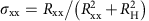is the longitudinal conductivity. The linearity of the curves over five orders of magnitude allows the description σxx(T) × T=σ0(B) exp[−(T0(B)/T)1/2] where T0(B) and σ0(B) are B-dependent fitting parameters, as expected from a dissipation mechanism based on VRH with soft Coulomb gap30 that has already been observed in exfoliated31,32 and epitaxial graphene19. Thermal activation does not manifest itself in the investigated temperature range up to 40 K. Figure 4b shows a sublinear increase of T0(B) as a function of B with a saturation around 2,500 K at the highest magnetic field. If we assume that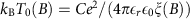where C=6.2 (refs 33, 34), kB is the Boltzmann constant,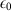is the permittivity of free space,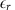is the mean relative permittivity of the graphene on SiC covered by the P(MMA-MAA) copolymer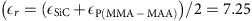, with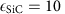(ref. 35) and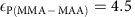is the value chosen, usually attributed to polymethylmethacrylate (PMMA) alone), then it is possible to determine the localization length ξ(B) as a function of the magnetic field B. Figure 4b shows that ξ(B) continuously decreases from ≈10.5 to ≈5.5 nm between B=7 and 19 T but does not show a minimal value. This continuous decrease of ξ(B) explains the robustness of the Hall resistance plateau towards high magnetic fields.Figure 4: Analysis of the dissipation based on the VRH mechanism.

It is also interesting to know whether the VRH mechanism can explain the dependence of Rxx on the current reported in Figs 2 and 5a. The VRH backscattering mechanism predicts that the current I manifests itself as an effective temperature Teff(I)=eRH/(2kBW) (ref. 34), where W is the sample width in the hypothesis of a homogenous electric field. It results that σxxexp[−(I0/I)1/2] at T=0 K, where I0=2kBT0W/(eRHξ) is a B-dependent current parameter. For several B values, the effective temperature Teff(I) is determined by matching σxx(Teff)=σxx(I), where σxx(I) is the conductivity measured as a function of the current and σxx(T)=(σ0/T)exp[−(T0/T)1/2] was determined previously from the data of Fig. 4a. For all B values, Fig. 5b shows a linear relationship between Teff and I as expected for the VRH mechanism. Moreover, the values of Teff extracted in the investigated current range, belongs to a range of low temperatures (<7 K) where the VRH was demonstrated to explain the behaviour of the longitudinal conductivity. From the slope of the Teff(I) curves and the previous determination of ξ(B), we can therefore extract an effective width Weff≈7.5 μm, quite independent of the magnetic field, which is much smaller than the Hall bar channel width W=100 μm. This indicates an inhomogeneity of the current flow in the sample, which holds up to large current values. In GaAs-QHRS supplied with high currents, several experiments based on the measurement of a linear dependence of the breakdown current of the QHE, as a function of the Hall bar width, have strongly supported a homogeneous distribution of the current36. On the other hand, sublinear behaviours were also observed, generally in higher carrier-mobility samples37,38. It turns out that the current distribution remains difficult to model because it is dependent on the microscopic details of the 2DEG, notably of the length scale of inhomogeneities34,36. In exfoliated graphene on SiO2/Si, it was shown, for example, that large fluctuations of the carrier density caused by the presence of charged impurities close in the substrate lead to a drastic reduction of the breakdown current of the QHE14. In our G-QHRS, Hall resistance measurements, performed at different places in the Hall bar do not reveal strong large-scale fluctuations of the carrier density (less than 10%). On the other hand, intermittent small bilayer patches existing along SiC edge steps constitute inhomogeneities that could constraint the flowing of the current across constrictions and favour the existence of large local electric fields, resulting in a reduced effective width Weff. Fortunately, being of small size compared with the sample width, these bilayer patches are not able to short-circuit the edge states, an extreme effect that has been modelled39,40 and recently observed in epitaxial graphene grown by sublimation of SiC41. The proof is the accuracy of the quantized Hall resistance, demonstrated with a 10−9-relative measurement uncertainty, in the two G-QHRS considered in this work.

The 2D colour plot of Fig. 2 gives a direct visualization of I(B) curves at constant longitudinal resistance values. They are sublinear, as highlighted by the black line that gives the evolution of the threshold current IC (which can be used to define a breakdown current of the QHE) above which Rxx>0.25 mΩ. IC(B) continuously increases from 40 to 60 μA for B varying from 10.5 to 19 T. This corresponds to breakdown current densities varying from 0.4 to 0.6 A m−1 if we assume, for the calculation, the 100-μm width of the channel in between voltage terminals used to measure Rxx. These values are similar to those measured in GaAs-QHRS but well below the best values reported in graphene grown by Si sublimation from SiC42. Nevertheless, we cannot omit that the injection of the current by the narrower I1 terminal of 20 μm width only could lead to a large underestimation of the breakdown current density. Furthermore, if we consider the effective width Weff=7.5 μm and the IC(B) values determined, we calculate higher breakdown current densities of 5.5 A m−1 at 10 T, 6.7 A m−1 at 14 T and 8 A m−1 at 19 T, in agreement with values expected in graphene. The sublinear evolution of IC as a function of B can also be explained by the VRH mechanism. Given that σxxexp[−(I0/I)1/2], we indeed expect a sharp increase of the conductivity for ICI0 with I0ξ−2 (at this critical current the tiny variation of σ0 becomes negligible). Figure 2 indeed shows that ξ−2(B) (red squares) well adjusts to the IC(B) (black line).

## Discussion

In graphene, the combination of a large energy gap between LLs, the existence of a LL at zero energy and a moderate carrier mobility, which ensures a large mobility gap, are favourable to a wide extension of the RK/2 Hall resistance plateau, well beyond the magnetic field corresponding to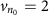. Such wide and asymmetric (with respect to the magnetic field giving ${v}_{{n}_{o}}\phantom{\rule{0.2em}{0ex}}\text{=}\phantom{\rule{0.2em}{0ex}}\text{2}$) RK/2 Hall resistance plateaus have even been reported in some works either in exfoliated graphene43 or in epitaxial graphene grown on the C-terminated face of SiC44. Their quantization properties were characterized by a minimum of the longitudinal resistance occurring at a magnetic field corresponding to. It results that an increase of the dissipation level, for example, caused by an increase of the measurement current, tends to restore a symmetric shape of both the Hall and the longitudinal resistance with respect to the magnetic field giving.

In the sample considered in this work, the magnetic field extension of the RK/2 Hall resistance plateau corresponds to a range of LL filling factor from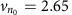(B=5 T) down to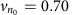(B=19 T) if a carrier density n0 constant with magnetic field is assumed. Moreover, theand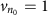LL filling factors should occur at B=6.6 T and B=13.2 T, respectively. Measurements of Rxx at a low current value (1 μA) as a function of B, reported in Fig. 6a reveals the existence of a tiny minimum that occurs at B≈15 T independently of the temperature between 1.3 and 40 K, but not at B=6.6 T, as would be expected in the hypothesis of a constant carrier density. On the other hand, ν=2 at B=15 T would mean a carrier density reaching 7.3 × 1011 cm−2 instead of n0=3.2 × 1011 cm−2. Moreover, this minimum is no more observable at larger currents of some tens of μA (see Figs 1c and 2) while the increase of the dissipation should, in principle, reinforce its existence. On the contrary, we observe an exceptionally wide Hall resistance plateau, which remains accurately quantized with regards to the 10−9-relative standard measurement uncertainty over a 9-T magnetic field range, for macroscopic currents of several tens of μA. This behaviour is not in agreement with observations reported in previously discussed works in refs 43, 44. On the other hand, it is rather similar to what was observed in epitaxial graphene grown on the Si-terminated face of SiC by Tzalenchuk et al.45: an asymmetric Hall resistance plateau extending towards large magnetic fields that stays robust, and quantized to RK/2 within a relative uncertainty of a few 10−9 over 2.5 T, at large measurement currents. It was notably characterized by a continuous increase of the breakdown current of the QHE well beyond the magnetic field corresponding to, as it is also observed in our sample (see Fig. 5a). This was explained by a pinning of the LL filling factor at ν=2 caused by a charge transfer from the zero layer graphene (ZLG), specific to the growth on the Si-face of SiC, existing at the interface between graphene and the substrate46.

To deepen our understanding, ξ(B), reported in Fig. 4b, was normalized by the magnetic length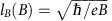, which describes the wavefunction characteristic size in the QHE regime. Figure 6b shows that ξ(B)/lB(B) goes down when increasing B up to 10 T, stays almost constant at a minimal value close to one between B=10 and 15 T and then slowly increases at higher magnetic fields. Let us remark that the determination of ξ(B) values slightly lower than the magnetic length, which was not expected, can be related to the assumptions regarding the values of the dielectric constants of SiC (the presence of the ZLG on SiC is not taken into account) and of P(MMA-MAA) (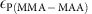could be slightly different from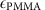), as well as of the C proportionality factor in the VRH expression of the conductivity (for instance, a larger value C=7.4 leads to ξ(B)lB and might result from the partial inhomogeneity of our two-dimensional system caused by the presence of bilayer patches). In the magnetic field range from 10 to 19 T, where the Hall resistance is accurately quantized with a 10−9-relative standard uncertainty, ξ(B) remarkably stays very close, within 10%, to lB(B). Figure 6c also shows that the dependencies of σ0(B) and ξ(B)/lB(B) on B are similar. It appears that the minimum of Rxx, observed in Fig. 6a at B≈15 T, occurs at the highest magnetic field for which both σ0(B) and ξ(B)/lB(B) have the lowest values.

In the QHE regime, the localization length ξ is expected to vary according to ξξ0/νγ (with γ≈2.3 (ref. 47) and ξ0 a length depending on the disorder potential) for ν≤2 and approaching ν=0. In samples made of exfoliated graphene, this law was observed32 to hold for ν values as high as 1.5. A lower bound value of ξ0 is lB, as predicted in case of short-range disorder48. We therefore expect ξ(B)/lB(B) higher than 1/ν(B)γ, which increases for decreasing ν values and then diverges at ν=0. For B varying from 10 to 15 T, although ${v}_{{n}_{o}}$(B) decreases from 1.3 down to 0.9, ξ(B)/lB(B) is observed to stay constant. This is a first indication that ν(B) might stay close to ν=2. Away from the LL centre near integer filling factors, it was proposed that ξ(B) should approach the classical cyclotron radius49. A localization length approaching rc=kF/eB at integer LL filling factor, where kF is the Fermi momentum, was indeed observed in GaAs-based 2DEG34. In graphene, rc(B) can be written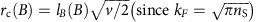. rc(B) is therefore proportional to lB(B) if ν(B) is constant (remarkably, one finds lB(B) for ν=2). The observation of ξ(B)lB(B) therefore constitutes another argument suggesting that ν(B) could be pinned at ν=2 from 10 to 15 T, and then decreases slowly up towards 19 T.

As discussed in Methods, structural characterization by low-energy electron diffraction shows the existence of a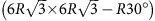reconstructed carbon-rich interface (ZLG) in our device24. Thus, a transfer of charges from the ZLG leading to a pinning of ν(B) at v=2 is possible and could explain the large width of the observed Hall resistance plateau, the absence of minima for both the localization length and the longitudinal conductivity at B=6.6 T. Using equations in refs 45, 46 derived from the balance equation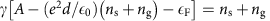describing the charge transfer, it is possible to reproduce a pinning at ν=2 from B=5.3 T up to B=15.1 T with a zero magnetic field carrier density of 3.2 × 1011 cm−2, considering A=0.4 eV, d=0.3 nm, γ=8.56 × 1012 cm−2 (eV)−1 and ng=1.6 × 1012 cm−2, where A is the difference between the work functions of undoped graphene and ZLG, d is the distance of the graphene layer to the ZLG, γ the density of donor states in ZLG and ng the density of carriers transferred to the electrochemical gate. This is rather consistent with the experimental observations except that the analysis of the dependence of ξ/lB on B rather indicates that the pinning of the filling factor should be effective at a higher magnetic field (B=10 T). Further experimental and theoretical works are needed to better understand the peculiarities of the charge transfer in graphene grown by propane/hydrogen CVD on SiC. Thereupon, the reduced effective width Weff over which the Hall potential drops, as determined from the analysis of the dissipation, could be an indication of some degree of inhomogeneity of the charge transfer.

To summarize, we report on the Hall resistance quantization of the ν=2 plateau in a sample made of graphene grown by propane/hydrogen CVD on SiC. The agreement with RK/2 of the quantized Hall resistance, measured with a 10−9-relative standard uncertainty (1 s.d.) at T=1.4 K, is demonstrated over a 9 T-wide magnetic field range extending from 10 to 19 T. Moreover, the relative discrepancy between the quantized Hall resistances in the graphene sample and in a reference GaAs one is equal to (−2±4) × 10−10. This constitutes a new proof of the universality of the QHE. The QHE physics of the wide quantized Hall resistance plateau is investigated using accurate specialized measurement techniques based on superconducting quantum interference device (SQUID) technology. From the characterization of the low dissipation, which is dominated by VRH, we determine that the localization length of states at Fermi energy stays locked to the magnetic length in the wide range of magnetic field where the Hall resistance is perfectly quantized. This can be explained by the pinning of the LL filling factor at ν=2 caused by a charge transfer from the buffer layer (ZLG) at the interface between the graphene and the SiC. The analysis of the dissipation caused by the current reveals that the Hall electric field in the QHE regime is inhomogeneous across the sample, which could be linked to the structure of graphene grown by propane/hydrogen CVD on SiC. A second G-QHRS from a different graphene growth, measured at T=1.3 K, is demonstrated to be 10−9 accurate at B=10 T and over a magnetic field range wider than in usual GaAs-QHRS. This argues for the reproducibility of the fabrication method of G-QHRS, which are able to substitute their GaAs counterparts, under the magnetic fields and low temperatures available in most national metrology institutes. This constitutes an essential step towards low magnetic field QHRS setting the basis of low-cost and transportable QHRS in the near future. Given that the propane/hydrogen CVD on SiC is a scalable growth technique that produces high-quality graphene meeting the demanding requirements of the resistance metrology, it is likely that it will be suitable for other electronic applications of graphene as well.

## Methods

### Graphene growth

Graphene was grown by propane/hydrogen CVD23,50 on the Si-face of a semi-insulating 0.16° off-axis 6H-SiC substrate from TanKeBlue. We used a horizontal hot-wall CVD reactor similar to that widespread in SiC electronic industry. A hydrogen/argon mixture (23% of hydrogen)25 at a pressure of 800 mbar was used as the carrier gas during the whole process. The graphene growth was obtained by adding a propane flow (0.04%) for 5 min at a growth temperature of 1,550 °C. Before the lithography, the graphene was extensively analysed (sample HT-MLG in ref. 24). Briefly, SiC steps of width 200 nm and height 0.75 nm were evidenced by atomic force microscopy24. Angle-resolved photoemission spectroscopy (ARPES) shows that a graphene monolayer covers the whole SiC surface, but ≈10% is covered by a second graphene layer (Fig. 7a,b). It grows discontinuosly and it is located mainly along SiC edge steps, forming small bilayer patches of no more than 300 nm in size. ARPES spectra also evidences high n-doping (1013 cm−2) of the graphene monolayer, whose origin can be linked to the presence of areconstructed carbon-rich interface detected by low-energy electron diffraction.

Finally, a remarkable homogeneity of the graphene film was evidenced in ref. 24 by the perfect superimposition of Raman spectra collected at different places of the sample. The lorentzian 2D peak and the normalized intensity of the G peak are typical of monolayer graphene. A notable D peak is observable but a large part originates for the underlying buffer layer24. The homogeneity of the graphene film is confirmed by the measurement of very similar electronic properties (carrier mobility, similar QHE) in the main Hall bar studied and another (third sample considered in this work) fabricated from a different piece (5 × 5 mm2 size) of the same graphene wafer (see Fig. 7c). Moreover, the structural properties of the graphene were demonstrated to be repeatable and well controlled by the growth parameters (pressure, temperature, propane and hydrogen flow)23,25,50. This is evidenced by the measurement of a second 10−9-accurate G-QHRS fabricated from a different graphene growth (several months later), as mentioned in subsection Resistance quantization.

### Sample fabrication

The graphene sample was annealed in vacuum () for 1 min at 500 °C (ramp of 500 s). The sample was left to cool down to below 100 °C in vacuum over a few minutes. Subsequently, it was covered with PMMA for protection. The Hall bars were patterned using electron-beam lithography with PMMA resist and oxygen reactive ion etching (RIE). Ohmic contacts to the graphene layer were formed by depositing a Pd/Au (60 nm/20 nm) bilayer in an electron-beam deposition system, using an ultrathin Ti layer for adhesion. Thicker Ti/Au (20 nm/200 nm) bonding pads were formed in a subsequent step, where a RIE etch was performed prior to metal deposition for better adhesion of the metal pads to the SiC substrate. The Hall bar has a width of 100 μm and a total length of 420 μm. It has three pairs of Hall probes, separated by 100 μm (see Fig. 1b and Fig. 8). Finally, the sample was covered for protection by 300 nm of poly(methylmethacrylate-co-methacrylate acid) copolymer (MMA (8.5) MAA EL10 from Microchem) and 300 nm of poly(methylstyrene-co-chloromethylacrylate) (ZEP520A from Zeon Chemicals) resist. The ZEP520A resist is known to reduce the electron density under ultraviolet illumination45,51. Nonetheless, no illumination was done in our case. Although not fully understood, the process leading to low carrier density is reproducible. Figure 7c, which reports the QHE in another Hall bar sample (third sample considered in this work) characterized by values of carrier mobility and density quite close to those of the main sample studied, illustrates the repeatability of the fabrication process of samples having a few 1011 cm−2 n-doping.

### Measurement techniques

The Hall resistance RH of a QHRS is compared with the 100-Ω resistance of a transfer wire resistor using a resistance bridge based on a CCC. The CCC is a perfect transformer that can measure a current ratio in terms of the winding number of turns ratio with a relative uncertainty as low as a few 10−11. Its accuracy relies on a flux density conservation property of the superconductive toroidal shield (Meissner effect), in which superconducting windings are embedded. Owing to a flux detector based on a direct current (d.c.) SQUID, the current noise resolution of the CCC is .

For measurements reported in Fig. 1a, the resistance bridge operates in d.c. mode (the current is reversed every 35 s) and is equipped with a EMN11 nanovoltmeter as a null detector. The QHR and the 100-Ω resistor are connected in series with a 2,065-turn winding and a 16-turn winding, respectively. Rxx is determined using an EMN11 nanovoltmeter to detect the longitudinal voltage Vxx resulting from the circulation of a d.c. current in the Hall bar.

For measurements reported in Figs 2 and 5a and for two measurements reported in the text (Resistance quantization section) carried out at B=10 and 19 T, the sample is biased with a d.c. current I and Rxx is measured using the CCC (see Fig. 8). A 2,065-turn winding of the CCC is connected to the two voltage terminals. The longitudinal voltage Vxx gives rise to the circulation of a current i in the winding of the CCC, which is used as a current amplifier with a SQUID operating in internal feedback mode. The output of the SQUID electronics is measured with an Agilent 3458A multimeter. The current noise resolution is 40 fA Hz−1/2, which results in a voltage noise resolution of ≈0.5 nV Hz−1/2. The longitudinal resistance Rxx is then given by Rxx=(i/I)RH since the two-terminal impedance seen by the winding is very close to RH on the ν=2 plateau.

For data reported in Figs 3 and 4, quick and accurate measurements are carried out while the temperature of the sample is swept from 40 K down to 3 K. The Hall bar is then supplied with an a.c. (2 Hz frequency) current I=1 μA, controlled by the reference voltage of a Signal Recovery 7265 lock-in detector. The Hall resistance RH is measured using the resistance bridge replacing the EMN11 nanovoltmeter used in d.c. to measure the voltage balance, by a Celians EPC1 a.c. low-noise amplifier whose output is connected to the lock-in detector. Rxx is measured using the CCC as in Figs 2 and 5a, except that the output of the SQUID is connected to the lock-in detector.